Understanding Geometry in IB Math
EDUCATION

# Understanding Geometry in IB Math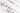Geometry is an essential part of the IB Math curriculum. It involves the study of shapes, sizes, and positions of objects, and it plays a vital role in many areas of mathematics and real-world applications. In this article, we will explore the basics of geometry, trigonometry, coordinate geometry, transformations, and their applications.

### Basics of Geometry

To begin, we need to define some key terms that we will be using throughout this article. A point is an exact location in space, and a line is a set of points that extend infinitely in both directions. An angle is formed when two lines or line segments intersect, and it is measured in degrees or radians.

A compass and straightedge are important tools used to construct geometric figures. A compass is a tool used to draw circles and arcs, while a straightedge is a tool used to draw straight lines. With these tools, we can construct various geometric shapes such as triangles, circles, and polygons.

Triangles are a fundamental shape in geometry. They are formed by connecting three points with line segments. A triangle’s angles add up to 180 degrees, and the sum of the lengths of any two sides is always greater than the length of the third side. A circle is a shape that is defined by a set of points that are equidistant from a fixed point called the center.

Polygons are closed shapes that have three or more sides. They can be regular or irregular, depending on whether all of their sides and angles are congruent or not. Examples of polygons include squares, rectangles, triangles, and pentagons.

### Trigonometry

Trigonometry is the study of the relationships between the sides and angles of triangles. It is a crucial part of geometry, and it is used in many real-world applications such as navigation, engineering, and physics. Trigonometric functions such as sine, cosine, and tangent are used to solve problems involving right triangles.

A right triangle is a triangle that has one angle measuring 90 degrees. The side opposite the right angle is called the hypotenuse, while the other two sides are called legs. The Pythagorean theorem states that in a right triangle, the sum of the squares of the legs is equal to the square of the hypotenuse.

The unit circle is a circle with a radius of one unit. It is used in trigonometry to find the values of sine, cosine, and tangent for any angle in radians. The unit circle is also used to find the values of inverse trigonometric functions such as arcsine, arccosine, and arctangent.

### Coordinate Geometry

Coordinate geometry is the study of geometric shapes using the Cartesian coordinate system. The Cartesian coordinate system is a grid made up of two perpendicular lines called the x-axis and y-axis. The point where the two axes intersect is called the origin.

In coordinate geometry, we use key terms such as slope, midpoint, and distance. The slope of a line is the ratio of the change in y-coordinates to the change in x-coordinates. The midpoint of a line segment is the point that is equidistant from both endpoints. The distance between two points is the length of the line segment that connects them.

We can use the distance formula and midpoint formula to solve problems involving geometric shapes in the Cartesian coordinate system. These formulas can help us find the length of a line segment, the coordinates of the midpoint, and the equation of a line given two points.

### Transformations

Transformations are operations that move or change the position of geometric shapes. They include translations, rotations, reflections, and dilations. A translation is a transformation that moves a shape horizontally and/or vertically. A rotation is a transformation that turns a shape around a fixed point. A reflection is a transformation that flips a shape across a line of reflection. A dilation is a transformation that enlarges or reduces the size of a shape by a certain scale factor.

When performing transformations, it is essential to understand the properties of the original shape and how they are affected by the transformation. For example, a translation does not change the size or shape of a shape, but it does change its position. A reflection changes the orientation of the shape, but it does not change its size or shape.

Congruent and similar figures are also important concepts in geometry. Two figures are congruent if they have the same size and shape, while two figures are similar if they have the same shape but different sizes. To determine if two figures are congruent or similar, we can use various methods such as side-angle-side (SAS), side-side-side (SSS), angle-angle (AA), and corresponding angles and sides.

### Applications of Geometry

Geometry has many real-world applications in fields such as architecture, engineering, and physics. Architects use geometry to design buildings and structures that are stable and aesthetically pleasing. Engineers use geometry to design and construct bridges, roads, and other infrastructure. Physicists use geometry to describe the motion of objects and to study the behavior of light and sound.

Geometry is also useful in everyday life. For example, if you need to hang a picture on the wall, you can use geometry to ensure that it is level and in the right position. If you want to calculate the area of a room or the volume of a container, you can use geometry formulas to make the calculations.

### Conclusion

Understanding geometry is crucial for success in IB Math and many other fields. By mastering the basics of geometry, trigonometry, coordinate geometry, transformations, and their applications, you can develop problem-solving skills that will be useful in various situations. Geometry is an essential tool for architects, engineers, physicists, and everyday people. Whether you are designing a building, constructing a bridge, or hanging a picture, geometry can help you achieve your goals with accuracy and precision.

## Recent Posts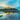TRAVEL

### Weekend Wanderlust: Unforgettable Getaways from Singapore by Ferry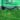HOME IMPROVEMENT

### Four Effective Tips to Maintain an Amazing Backyard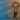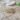HEALTH

### 5 Interesting Ways in Which Soybeans Are Considered Healthy

#### Related Articles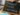EDUCATION

### 10 Benefits of Project-Based Learning

Are you looking for an international school for your child? Even at...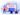EDUCATION

### What is the name of the OpenShift certification?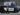EDUCATION

### 5 Certifications You Must Have to Become a First Responder

The path of becoming a first responder represents an esteemed and indispensable...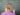EDUCATION

### How many years of Spanish does it take to be fluent?

In an increasingly interdependent world, mastering multiple languages is an invaluable asset,...